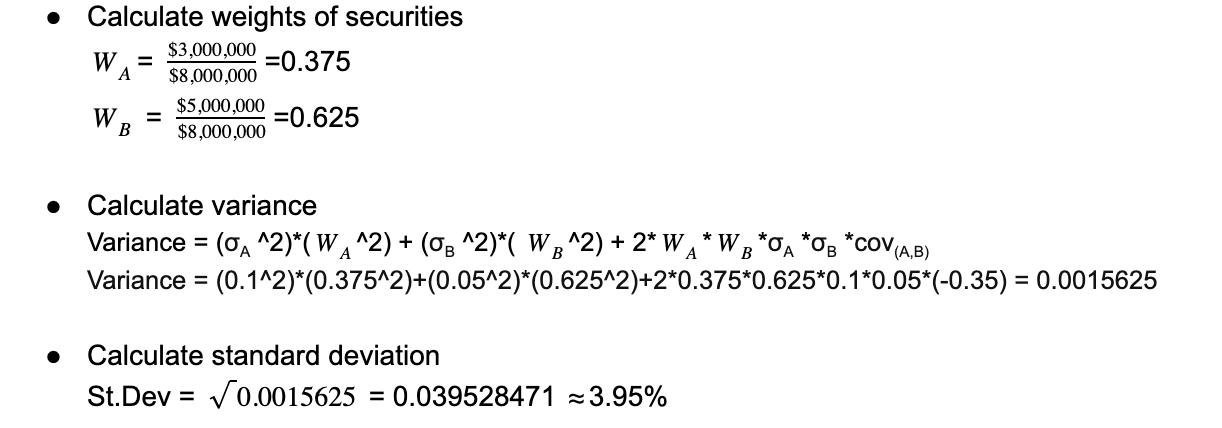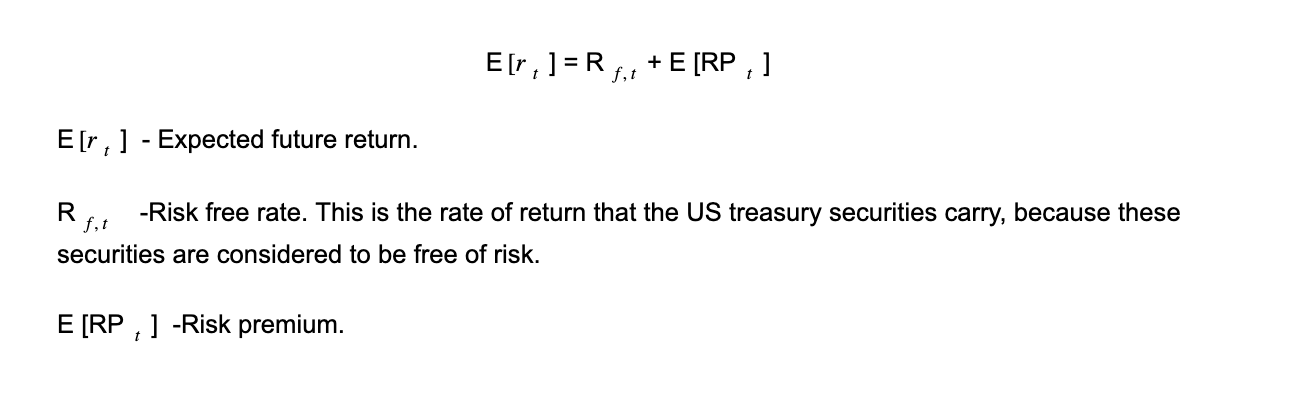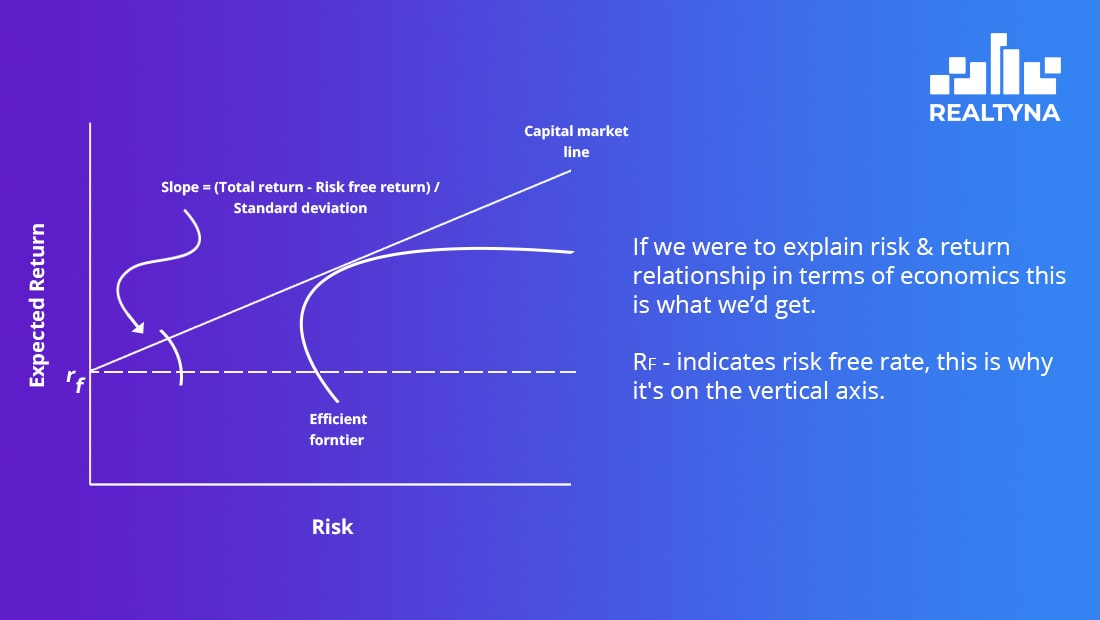# Risk & Return on Real Estate Investment

The very backbone of finance is the concept of the risk and return relationship. Should come as no surprise that the higher the risk, the greater the return, and conversely, lower risk gets you lower return.

But the main question here is how one should examine the exact relationship between the two? How can we find the exact point where risk is minimized and return – maximized? Where lies the best option? How does one calculate risk of investments in real estate? And this is just the tip of the iceberg.

### BREAKING DOWN STANDARD DEVIATION

Standard deviation is just a fancy statistics/finance word for risk.
Think of standard deviation as a dispersion measurement for returns of financial assets which belong to the same investment portfolio. The higher the standard deviation of a portfolio the riskier it is overall. For instance, if you are not sure about the monthly returns that certain real estate investment units would provide you with, then that accounts for high volatility for your portfolio.
However, we still deal with risk as a finance concept when it comes to individual investments as well.
Investors tend to be risk averse. If they’re given two investment possibilities with the same future expected returns and different risks associated with them, then they’d of course go for the one which comes with the lower risk. No-brainer. But it gets much more complex when risks and returns are both different.

### HOW TO CALCULATE STANDARD DEVIATION OF A PORTFOLIO

Consider a case where we have 2 different securities (real estate investments) under one portfolio. And the total investment is on amount of \$8,000,000. Let’s make an assumption that the following is true:

We also know that correlation, aka cov(A,B), between the two investments is -0.35, meaning, that if one security increases/decreases by \$1, another one will decrease/increase by \$0.35. Note that the correlation is negative.

Okay now we can go ahead and calculate the standard deviation of the portfolio as follows:Notice that 3.95% is basically the risk level of a portfolio and it is less than the original individual risk level of either securities: 10% and 5%. Thus, by investing in two assets with negative correlation, we’ve diversified our investment and reduced the overall risk level.

As you can see, in terms of real estate, diversification can be made possible if investors distribute the investment among different types of properties (residential, commercial, industrial…) and it would also be of help to diversify the portfolio with properties from completely different parts of the city, such as CBDs & suburbs.

Now that we know not all investments are equal in terms of the risk they carry, it makes sense that they should provide different returns to investors, or else – such investments are non-feasible to invest in. Here’s where risk premium steps in, in order to balance the equation.In real estate, however, cap rate is what investors usually compare to the returns of other investments in order to evaluate the feasibility of this or that property. For example, if an investor has to make a decision between investing in a certain property and investing in some company’s bond, he/she would have to compare the property’s cap rate to the return rate of the bond. The higher the cap rate the better for the investors. If it’s higher than the return on the bond, investors most probably would invest in the property. To find out more about cap rate in real estate see What is CAP Rate in Real Estate?

### WHERE RISK FREE RATE COMES FROM

By investing in risk free asset, investors can be sure return will be equal to risk free rate. For instance, if you made a risk free investment for \$100 and at the end of the year your return was \$110, then the risk free rate was 10%. Now, this rate is solely there to compensate the investors for Time Value of Money – a dollar today is worth more than a dollar in one year. This is also known as one of the fundamental rules of finance.

### PORTFOLIO DIVERSIFICATION

You may have heard this saying about not putting all the eggs in one basket, right? This idea is the very foundation of portfolio diversification theory. This means that a good investor should always invest in different assets carrying significantly different characteristics. Ceteris paribus, assets that tend not to “move together” with the rest of the assets in the portfolio are better investment, than those that tend to follow the movement of other assets.

### Why?

Because such assets always reduce the volatility of a portfolio. This stands even if the asset itself has a considerably high volatility itself.
Thus, risk level of an asset is not the one and only decision-making condition, but rather, in order to maximize expected return, good investors should also consider co-movement of the asset relative to the rest of the assets in the portfolio.
Portfolio diversification theory is what makes internationally diversified portfolios so popular nowadays. I mean is there any better way of acquiring portfolio diversification than making sure you invest in different countries (continents even)? This is known as Modern Portfolio Theory.

### BOTTOM LINE

In case you’ve made it till here and managed not to get bored with all the real estate finance talk, you might want to take a look at some of our other finance-related articles as well: What is Natural Vacancy In Real Estate & What Factors Influence Home Value? Got a burning question? Drop us a line down below.</span

Rate our blogs